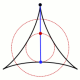2:16 AM
A new tag . Already with 16 questions.
7A real matrix $M$ is called totally positive if all of its minors are positive; these matrices have been extensively studied, and there are generalizations to other Lie types, for example by Lusztig. I am interested in the following $q$-analog: a matrix $M$ with entries in $\mathbb{R}[q]$ is $q$...

7The (twisted) cyclic shift $(v_1,v_2,\ldots,v_n) \mapsto (v_2,v_3,\ldots,v_n,(-1)^{k-1}v_1)$ acting on the Grassmannian $\mathrm{Gr}(\mathbb{C};k,n)$ of $k$-planes in $\mathbb{C}^n$ is an important symmetry with applications to combinatorics, geometry, representation theory, et cetera, especially...

13A totally positive matrix $M\in \mathcal{M}_{n\times m}(\mathbb R)$ is such that all of its minors of all sizes are positive. It is true that any Vandermonde matrix (with well-ordered positive entries) is totally positive. It seems that this fact should be classic. Although I can prove it by a va...

3Let $S$ be the set of $3 \times 3$ matrices $A$ satisfying the following conditions: All minors are $>0$ (i.e., $A$ is a strictly totally positive matrix); all principal minors are $>1$, except for the $3\times 3$ minor $\mathrm{det}(A)$, which is assumed to be $1$. By general theory of total...

10A matrix of real numbers is called totally positive if all its minors are non-negative. A well-known example is the Pascal matrix $(\binom{i}{j})$. Is it true that the minors of the $q$-Pascal matrix $({\binom{i}{j}}_q)$ are polynomials with non-negative coefficients?

5I was reading Postnikov's paper [TOTAL POSITIVITY, GRASSMANNiANS, AND NETWORKS] when I came across the definition of the totally nonnegative Grassmannian $Gr_{kn}^{tnn} \subset Gr_{kn}$ as the quotient $Gr_{kn}^{tnn} = GL_k^{+}\backslash Mat^{tnn}_{kn}$, where $Mat^{tnn}_{kn}$ is the set of...

2Recently I begin working on matroids, in particular to a generalization of oriented matroids to the complex case. I found on arxiv the following interesting articles: 1)Alexander Postnikov: Total positivity, Grassmanians and networks 2)Federico Ardila, Felipe Rincón, Lauren Williams: Positiv...

2What is the symmetry group of the totally nonnegative Grassmannian $Gr_{tnn}(k,n)$? [The latter consists of those elements of the Grassmannian that can be represented by $k \times n$-matrices all of whose $k \times k$-minors have non-negative determinants and $k < n$.]

9I'm looking at this matrix: $$\begin{pmatrix} 1 & 1/2 & 1/8 & 1/48 & 1/384 & \dots \\ 0 & 1/2 & 1/4 & 1/16 & 1/96 & \dots \\ 0 & 0 & 1/8 & 1/16 & 1/64 & \dots \\ 0 & 0 & 0 & 1/48 & 1/96 & \dots \\ 0 & 0 & 0 & 0 & 1/384 & \dots \\ \vdots & \vdots & \vdots & \vdots & \vdots & \ddots \end{pmatrix}... 16I am looking for information on the mathematician Anne Marie Whitney. She wrote a number of significant papers related to total positivity with her thesis adviser Isaac Schoenberg. All I could find on the internet is https://www.genealogy.math.ndsu.nodak.edu/id.php?id=36202. 5Decorated permutations are defined as permutations where the fix-points come in two colors (say \overline{\cdot} and \underline{\cdot}). For example, the 16 decorated permutations of length 3 are$$\overline{1}\overline{2}\overline{3},\underline{1}\overline{2}\overline{3},\overline{1}\underli...

68The paper ”Scattering Amplitudes and the Positive Grassmannian” by Nima Arkani-Hamed, Jacob L. Bourjaily, Freddy Cachazo, Alexander B. Goncharov, Alexander Postnikov, and Jaroslav Trnka, introduces a remarkable new way for computations in quantum field theory based on volume computations of cer...

66Is it possible to appreciate the geometric/polytopal properties of the amplituhedron without delving into the physics that gave rise to it? All the descriptions I've so far encountered assume familiarity with quantum field theory, but perhaps there exist more purely geometric explications...? If ...

3What are the roles that the classic number arrays-- Eulerian, Narayana--play in the application of totally non-negative Grassmannians, or amplituhedrons, to string / twistor scattering theory? (This is a severely reduced version of the original post, which can be found at my blog https://tcjpn.w...

2In the paper The Amplituhedron , Nima Arkani-Hamed and Jaroslav Trnka introduced the geometric object amplituhedron. It is defined as follows (see also the lecture notes). Let $Z$ be a $(k+m)\times n$ real matrix with maximal minors positive. Let $\tilde{Z}: Gr_{k,n}^{\geq 0} \to Gr_{k,k+m}$ be...

4In the lecture notes, it is said that (Theorem 3.1.3) the set of positroid cells in $Gr(k,n)$ are in one to one correspondence with the set of bounded affine permutations of type $(k,n)$. In Example 4.1.5, it is said that the permutation $\sigma$ corresponds to the big cell $Gr(3,6)$. What are th...

13 hours later…3:41 PM
@MartinSleziak Since I got no response, I have edited the two question manually. Unless it is used elsewhere the tag should be gone by tomorrow. mathoverflow.net/posts/346841/revisions mathoverflow.net/posts/347334/revisions
I see that the tag with this name was created before in 2016, the tags were edited by Mikhail Katz back then: mathoverflow.net/posts/243126/revisions
Another tag created in the same question is . The question whether or not this tag is going to be useful remains.